# Magic square of squares

Allen William Johnson  discovered the following magic square whose entries are all squares.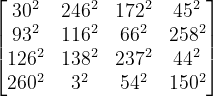The following Python code verifies that this is a magic square.

    import numpy as np

M = np.array(
[[ 30**2, 246**2, 172**2,  45**2],
[ 93**2, 116**2,  66**2, 258**2],
[126**2, 138**2, 237**2,  44**2],
[260**2,   3**2,  54**2, 150**2]
])

def verify(M):

m, n = M.shape
assert(m == n)

c = sum(M[0, :])
semimagic = True
for i in range(m):
semimagic &= sum(M[i,:]) == c
semimagic &= sum(M[:,i]) == c

d1 = sum(M[i, i  ] for i in range(m))
d2 = sum(M[i,-i-1] for i in range(m))
magic = semimagic and (d1 == d2 == c)

if magic:
return "magic"
if semimagic:
return "semi-magic"
return "not magic"

print(verify(M))


## More magic square posts

 Allen William Johnson. Journal of Recreational Mathematics. 22 (1990), 38

Here are three things about dominoes, two easy and one more advanced.

## Counting

First, how many pieces are there in a set of dominoes? A domino corresponds to an unordered pair of numbers from 0 to n. The most popular form has n = 6, but there are variations with other values of n. You can show that the number of dominoes isThis is because there are n+1 possible numbers (since blanks are a possibility) and each one is either a double or not. The number of ways to choose two distinct numbers is the binomial coefficient and the number of doubles is n+1.

Another way to look at this is that we are selecting two things from a set of n+1 things with replacement and so the number of possibilities iswhere the symbol on the left is Stanley’s symbol for selection with replacement.

In any case, there are 28 dominoes when n = 6, 55 when n = 9, and 91 when n = 12.

## Magic squares

There are a couple ways to make a magic square of sorts from a set of dominoes. To read more about this, see this post.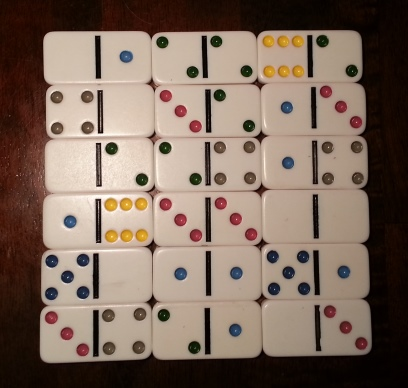## Tiling

How many ways can you cover an m by n chess board with dominoes? The answer turns out to beSee this post for details.

# Magic squares as matrices

If you view a 3 × 3 magic square as a matrix and raise it to the third power, the result is also a magic square.

More generally, if you multiply an odd number of 3 × 3 magic squares together, the result is a magic square.

For example, here are three magic squares that appeared in my blog post on Spanish magic squares, and you can verify that their product is another magic square.Source: Martin Gardner, Some New Discoveries About 3 × 3 Magic Squares, Math Horizons, February 1998.

The same article conjectures that the results above are true for magic squares of any odd order. That was 20 years ago. Maybe the conjecture has been resolved by now. If you know, please leave a comment.

# Consecutive pair magic square

The following magic square has a couple unusual properties. For one, numbers appear in consecutive pairs. Also, you can connect the numbers 1 through 32 in a continuous path.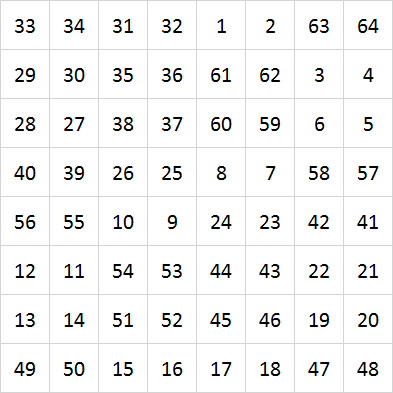I found this in Before Sudoku. The authors attribute it to William Mannke, “A Magic Square.” Journal of Recreational Mathematics. 1 (3) page 139, July 1968.

Other magic squares:

# Magic hexagon

The following figure is a magic hexagon: the numbers in any straight path through the figure add to 38, even though paths may have length three, four, or five.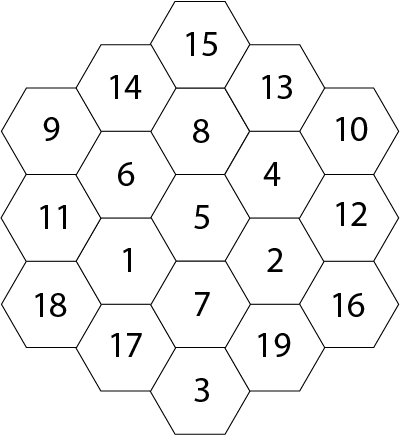I found this in Before Sudoku. The authors attribute it to Madachy’s Mathematical Recreations.

This is essentially the only magic hexagon filled with consecutive integers starting with one. The only others are rotations or reflections of this one, or the trivial case of a single hexagon.

# Magic square rows and columns as numbers

Take any 3 by 3 magic square. For example, here’s the ancient Lo Shu square: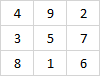If you read the rows as numbers and sum their squares, you get the same thing whether you read left to right or right to left. In this case

4922 + 3572 + 8162 = 2942 + 7532 + 6182.

Similarly, if you read the columns as numbers and sum their squares, you get the same thing whether you read top to bottom or bottom to top:

4382 + 9512 + 2762 = 8342 + 1592 + 6722.

This doesn’t depend on base 10. It’s true of any base. And the entries of the magic square do not have to be single digits as long as you take the first to be the coefficient of b2, the second the coefficient of b, and the last the coefficient of 1, where b is your base.

In addition to rows and columns, you can get analogous results for diagonals.

4562 + 9782 + 2312 = 6542 + 8792 + 1322

4562 + 3122 + 8972 = 6542 + 2132 + 7982

2582 + 9362 + 4712 = 8522 + 6392 + 1742

2582 + 7142 + 6932 = 8522 + 4172 + 3962

How would you prove this? Arthur Benjamin and Kan Yasuda give an elegant proof here using permutation matrices. Or you could use brute-force starting with Édouard Lucas’ theorem that every 3 by 3 magic square has the following form.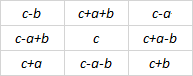(For each ab, and c there are eight variations on magic square given by Lucas, reflections and rotations of his square.)

Benjamin and Yasuda attribute this discovery to R. Holmes in 1970. “The magic magic square”, The Mathematical Gazette, 54(390):376.# Alphamagic squares in French

In earlier blog posts I give examples of alphamagic squares in English and in Spanish. This post looks at French.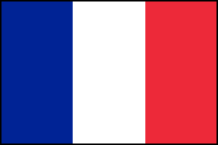The Wikipedia article on alphamagic squares, quoting The Universal Book of Mathematics, says

French allows just one 3 × 3 alphamagic square involving numbers up to 200, but a further 255 squares if the size of the entries is increased to 300.

My script did not find a French alphamagic with entries no larger than 200, but it did find 254 squares with entries no larger than 300. (For each of these squares there are 7 more variations you can form by rotation and reflection.)

The French alphamagic square with the smallest maximum entry that I found is the following.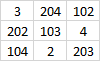When spelled out in French we getAnd when we replace each cell with its number of letters we get the following magic square: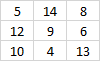A list of all the French magic squares with maximum element 300 that I found is available here.

# Alphamagic square in Spanish

In a previous post I gave an example of an alphamagic square in English. This is a magic square such that if you replace each number with the letter count when spelling out the word, you get another magic square.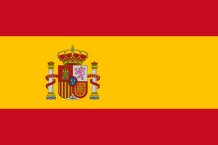I wondered whether I could find an alphamagic square in Spanish, so I wrote a script to look. I found two. (Plus there are eight rotations and reflections of each.)

The first is the following: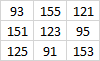When spelled out in Spanish the numbers are: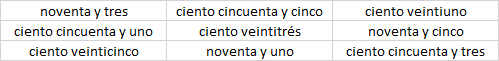And the number of letters in each cell gives: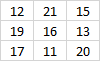Here’s a second example: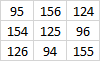Spelled out in Spanish: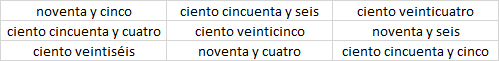Sum of the letters: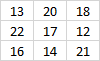If my script is correct, these are the only examples (besides their rotations and reflections) for numbers between 1 and 200.

# An alphamagic square

British engineer Lee Sallows came up with the following unusual magic square.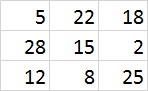If you spell out the English name of each numberthen replace the contents of each cell with the number of letters inside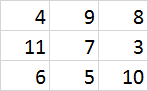you get another magic square!

Update: Here are a couple alphamagic squares in Spanish and more in French.

# Magic square made of dominoes

You can arrange a standard set of dominoes into a magic square of sorts. There are 28 dominoes, each with two ends, so the number of ends isn’t a perfect square. But if you ignore the row of blanks at the bottom, you have a 7 by 7 square where every row, column, and diagonal add to 24. (Technically this isn’t a magic square since some numbers repeat.)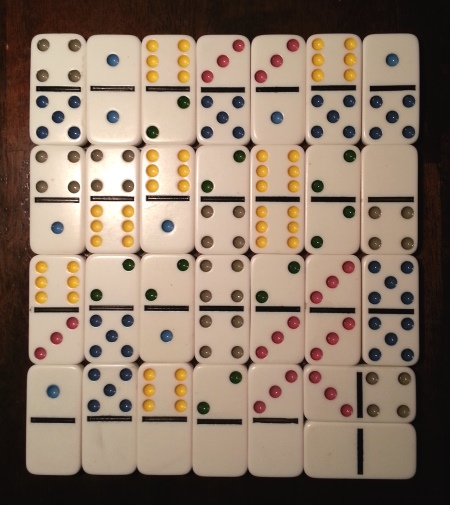If you don’t use all the dominoes you can make an actual square, no extra row to ignore:I found the design of these magic squares in The Zen of Magic Squares, Circles, and Stars by Cliff Pickover. He credits Mathematical recreations and essays by Ball and Coxeter.

While the most common set of dominoes goes up to double six, there are other possibilities. I made the images above by pulling out the double six subset out of a set of double nine dominoes. Is it possible to make magic squares like the first photo above out of a full set of dominoes up to double n for other values of n? Not if n is odd, but perhaps if n is even.

You can at least arrange the dominoes into a square. A set of double n dominoes has (n+1)(n + 2)/2 pieces, so (n+1)(n + 2) squares. With a row of n + 1 blanks on the bottom, this leaves (n + 1)2 squares in the magic square. The total number of points in a set of double n dominoes is n(n + 1)(n + 2)/2, so each row, column, and diagonal would have to sum to n(n + 2)/2. This means you cannot arrange a set of double n dominoes into a magic square if n is odd. So, for example, it won’t work for double nine dominoes. Is it possible when n is even? Certainly if n = 6, but what about other values of n?